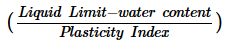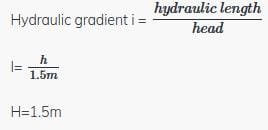# Test: Soil Mechanics - 2

## 25 Questions MCQ Test Civil Engineering SSC JE (Technical) | Test: Soil Mechanics - 2

Description
Attempt Test: Soil Mechanics - 2 | 25 questions in 50 minutes | Mock test for Civil Engineering (CE) preparation | Free important questions MCQ to study Civil Engineering SSC JE (Technical) for Civil Engineering (CE) Exam | Download free PDF with solutions
QUESTION: 1

Solution:
QUESTION: 2

Solution:
QUESTION: 3

### Uniformity coefficient of a soil is

Solution:
QUESTION: 4

According to Atterberg, the soil is said to be of medium plasticity if the plasticity index PI is

Solution:

because 0-7 :Low, 7-17 : Medium, >17 : High Plasticity.

so for soil is said to be of medium plasticity if the plasticity index PI is : 7

In general, 7 is the answer because it is on the lower end.

QUESTION: 5

If the natural water content of soil mass lies between its liquid limit and plastic limit, the soil mass is said to be in

Solution:
QUESTION: 6

When the plastic limit of a soil is greater than the liquid limit, then the plasticity index is reported as

Solution:
QUESTION: 7

The ratiofor a soil mass is called

Solution:
QUESTION: 8

Toughness index is defined as the ratio of

Solution:
QUESTION: 9

If the plasticity index of a soil mass is zero, the soil is

Solution:
QUESTION: 10

The admixture of coarser particles like sand or silt to clay causes

Solution:
QUESTION: 11

Select the correct statement.

Solution:
QUESTION: 12

The water content of soil, which represents the boundary between plastic state and liquid state,is known as

Solution:
QUESTION: 13

Which of the following soils has more plasticity index?

Solution:
QUESTION: 14

At liquid limit, all soils possess

Solution:
QUESTION: 15

According to IS classification, the range of silt size particles is

Solution:
QUESTION: 16

Highway Research Board (HRB) classification of soils is based on

Solution:
QUESTION: 17

Dispersed type of soil structure is an arrangement comprising particles having

Solution:
QUESTION: 18

Effective stress is

Solution:

Effective stress is a defined property which cannot be measured and is calculated by measuring the total stress and pore water pressure in the field.

Effective Stress= Total Stress- Pore water Pressure

It is not the true contact stress between particles because it uses area for division as gross area rather than Area of actual contact between particles

QUESTION: 19

The degree of saturation in soils can be defined as the ratio of

Solution:
QUESTION: 20

Rise of water table above the ground surface causes

Solution:
QUESTION: 21

The total and effective stresses at a depth of 5 m below the top level of water in a swimming pool are respectively

Solution:
QUESTION: 22

If the water table rises upto ground surface, then the

Solution:
QUESTION: 23

The critical hydraulic gradient ic of a soil mass of specific gravity G and voids ratio e is given by

Solution:
QUESTION: 24

The hydraulic head that would produce a quick condition in a sand stratum of thickness 1.5 m,specific gravity 2.67 and voids ratio 0.67 is equal to

Solution:

G=2.67,e=0.67QUESTION: 25

Physical properties of a permeant which influence permeability are

Solution:Use Code STAYHOME200 and get INR 200 additional OFF Use Coupon Code